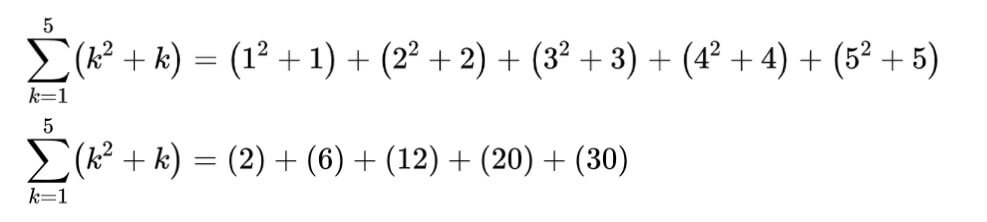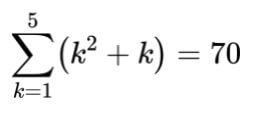# Sum of Series Calculator

Input the value of k (sequence) and n (number of terms) in the sum of a series calculator.

Give Us Feedback

A series sum calculator is a tool devised to add the values of a series. On giving n it finds all of the n’s till that number and adds them. You can add a series of up to 9 terms or even 1000 terms using this calculator.

## What is a series?

Series is the same as sequence and grows with a certain pattern. The only difference between sequence and series is that in series, we have a plus (+) symbol between the entries instead of a comma.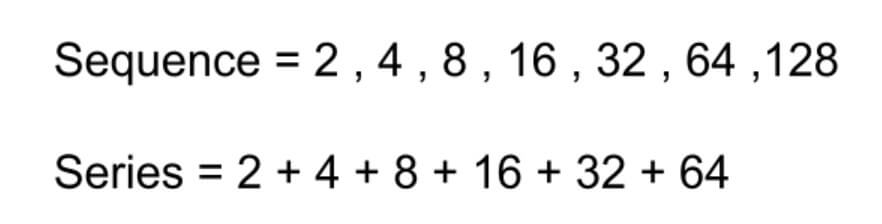## How to find the sum of a series?

To sum a series, we represent it as: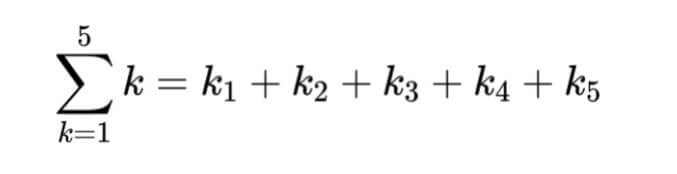Here,

n is the number of terms also known as the upper limit.

K = 1 is referred to as starting value.

ki can be any function for example k2, (k+1)-(k3) e.t.c.

In the series sum calculator above, the starting value is 1 by default.

Example

Upper limit n = 5

Starting value = 1

Function = k2+k

Solution:

Step 1: Write the series.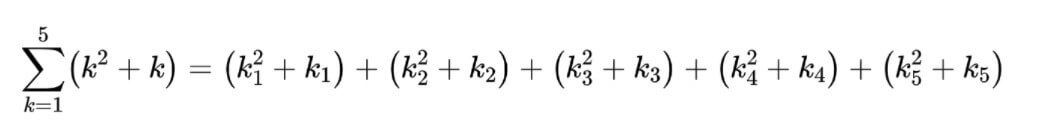Step 2: Put the values.